## EFFECTS OF THE ADDED CYLINDERS WITH DIFFERENT CONTROL ANGLES ON THE VORTEX-INDUCED VIBRATIONS OF A CIRCULAR CYLINDER 1)

Chen Weilin, Ji Chunning2), Xu Dong

State Key Laboratory of Hydraulic Engineering Simulation and Safety, Tianjin University, Tianjin 300072, China

 基金资助: 国家自然科学基金.  51621092国家自然科学基金.  51579175国家自然科学基金.  51779172NSFC-广东联合基金(第二期).  U1501501

Received: 2018-09-27   Accepted: 2018-10-18   Online: 2019-03-18

2)及春宁,教授,主要研究方向：流固耦合,涡激振动,泥沙运动.E-mail：cnji@tju.edu.cnAbstract

Vortex-induced vibrations of an elastically mounted circular cylinder will be altered through influencing the development of the boundary layer of the surface and the vortex shedding by the added smaller cylinders. The excitation or suppression of vortex-induced vibrations can be obtained by changing the arrangement and number of the small cylinders. In the former, more fluid energy can be transformed into mechanical energy or electricity while the latter can be applied to protect the structures. Numerical simulations of a transversely vibrating cylinder with two small cylinders behind were conducted, where the Reynolds number is 100, based on the main cylinder, the mass ratio is 2.0 and the reduced velocity is 3~11. The diameter ratio between the small and the main cylinder is 0.125 and the gap ratio is 0.125. Results indicate that the small cylinders can change the vibration of the main cylinder significantly in the simulated control angle range of 30°~90°. When the control angle is small (30°), the small cylinder suppresses the vibration of the main cylinder. The response can be divided into two branches, i.e. VIV-and galloping-branch, at the control angle of 45°~60°. The vibration amplitude increases monotonically with the increasing reduced velocity in the galloping branch. When the control angle is large (75°~90°), the promotion from the small cylinder decreases with the increase of the control angle. Furtherly, mechanisms of the small cylinders are explained by combining vortex shedding and pressure distribution around the cylinder of different instants in one period. Analysis of the energy coefficient indicates that the energy transferred from the fluid to the main cylinder decreases with the reduced velocity, which is caused by the variation of vortex structures.

Keywords： vortex-induced vibration ; immersed boundary method ; small cylinder ; control angle ; galloping

Chen Weilin, Ji Chunning, Xu Dong. EFFECTS OF THE ADDED CYLINDERS WITH DIFFERENT CONTROL ANGLES ON THE VORTEX-INDUCED VIBRATIONS OF A CIRCULAR CYLINDER 1). Chinese Journal of Theoretical and Applied Mechanics[J], 2019, 51(2): 432-440 DOI:10.6052/0459-1879-18-208

## 引 言

Muddapa和Patnaik研究发现,在参数组合(雷诺数$Re = 100$、直径比d/D = 0.1、控制角$\theta$ = 120$^\circ$,无量纲转速$\alpha = 2.0$)条件下,主圆柱的脱涡受到完全抑制.李椿萱等对附属小圆柱对主圆柱绕流的研究发现,不论小圆柱个数如何,总是存在一个最优的抑制区域.此时,涡街被完全抑制,阻力显著下降.Jiménez-González和Huera-Huarte的实验结果表明(雷诺数$Re$ = 9.2×10$^{3}\sim$2.6×10$^{4}$、直径比d/D = 0.12、质量比$m^{ \ast }$ = 1.94、阻尼比$\zeta$ = 0.011 7,参数定义见1.2节),当$\theta$ = 160$^\circ$和G/D = 0.045时,涡激振动抑制效果最佳,圆柱振幅下降了66%;而当$\theta$ = 90$^\circ$时,控制圆柱对主圆柱的振动有促进作用.此外,与振幅不同,脱涡频率对小圆柱的位置并不敏感.Zhu和Gao的数值结果表明(雷诺数$Re=3484$、 直径比d/D = 0.06、控制角为$\theta$ = 135$^\circ$、间隙比为G/D = 0.09),内向反转小圆柱向主圆柱边界层输入动量,使边界层分离延迟,分离点后移,抑制主圆柱振动;相反地,外向反转小圆柱使主圆柱的振幅增加.Korkischko和Meneghini实验研究了雷诺数$Re$ = 1.6×10$^{3}$~7.5×10$^{4}$下两个小圆柱对主圆柱涡激振动的影响情况. 其中,质量比$m^{ \ast }$ = 1.8、阻尼比$\zeta$ = 0.01、直径比d/D = 0.06、间隙比G/D = 0.07、控制角$\theta$ = 90$^\circ$、无量纲转速$\alpha$ = 5~10. 研究结果表明,与无附加小圆柱工况相比,当$\alpha$ = 5~10时,圆柱的振幅下降超过了57%,且抑制出现在较大的折合流速范围内.

## 1 数值方法

### 1.1 控制方程

$$\frac{\partial {u}}{\partial t} =-\left( {{{u}} \cdot \nabla } \right){{u}}-\nabla p + \nu \nabla ^2{{u}} + {{f}}$$

$$\nabla\cdot {{u}} = 0$$

$m\frac{{\rm d}^2y}{{\rm d}t^2} + c\frac{{\rm d}y}{{\rm d}t} + ky = F_y$

### 图1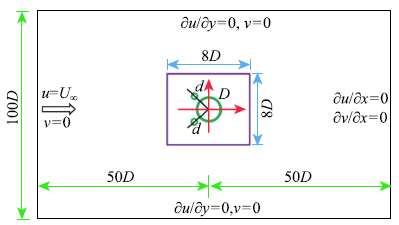Fig.1   The computational domain and boundary conditions

## 2 程序验证

Table 1  Comparison of vortex-induced vibrations of an isolated cylinder

W/DCDCL,maxSt
Ref.0.572.080.880.188
Ref.0.582.220.770.196
present0.572.090.750.185

## 3 结果和讨论

### 图2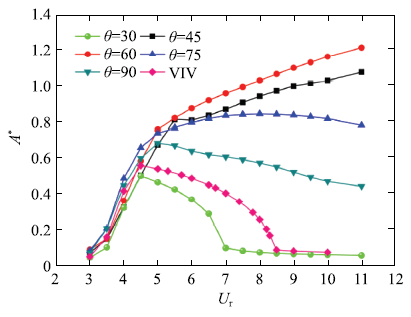Fig.2   Vibration amplitude versus the reduced velocity under different control angles

### 图3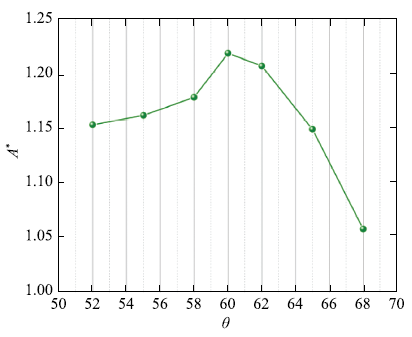Fig.3   Vibration amplitude varies with the control angle at$U_{\rm r}= 11$

### 图4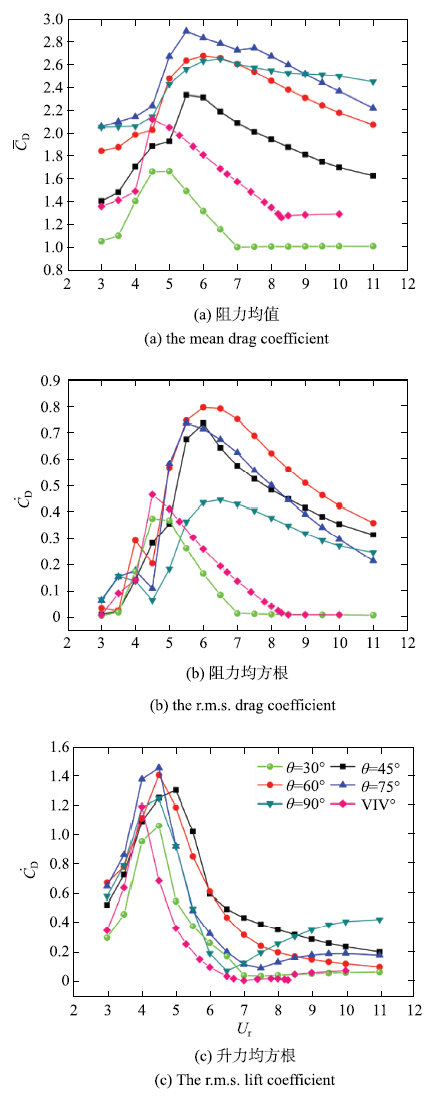Fig.4   Fluid forces versus the reduced velocity at different control angles

### 图5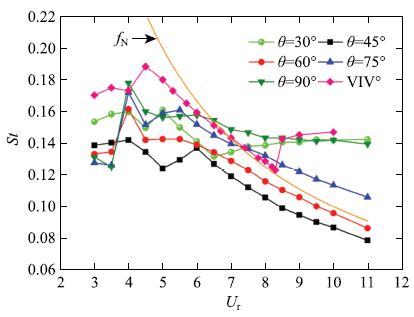Fig.5   Vortex shedding frequency versus the reduced velocity at different control angles

### 图6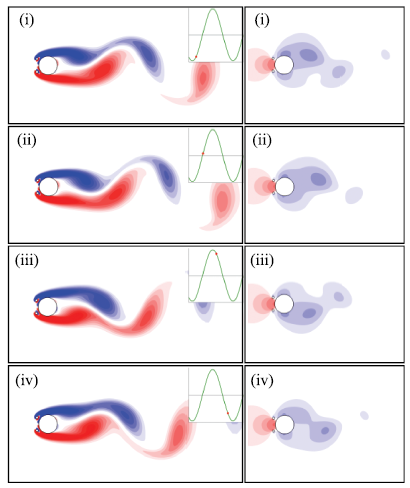Fig.6   Vorticity fields (left) and pressure fields (right) around the circular cylinder at$U_{\rm r} = 11.0$ and$\theta = 30^ \circ$

### 图7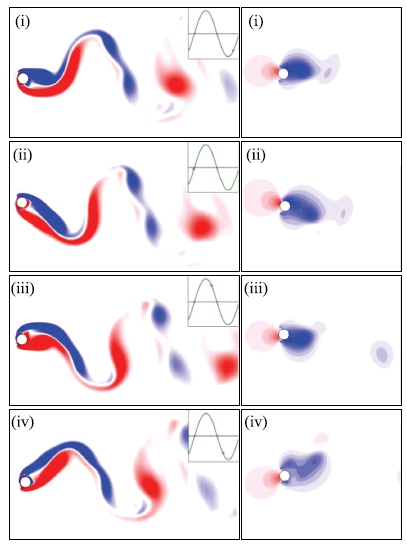Fig.7   Vorticity fields (left) and pressure fields (right) around the circular cylinder at$U_{\rm r} = 11.0$ and$\theta = 60^ \circ$

### 图8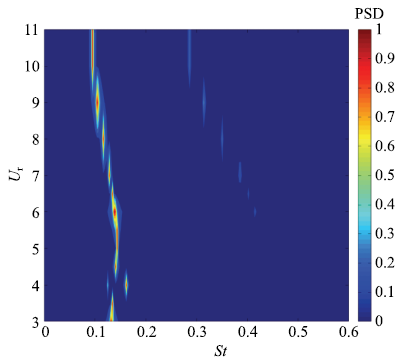Fig.8   Lift spectra of the main cylinder versus the reduced velocity at$\theta = 60^ \circ$

### 图9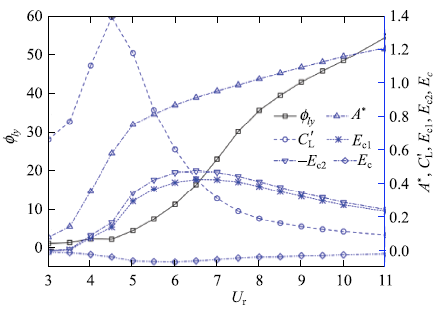Fig.9   The phase difference between the lift and displacement ($\varphi _{ly} )$, the r.m.s. lift coefficient ($C'_{\rm L} )$, the vibration amplitude ($A^ * )$ of the main cylinder, and energy transfer coefficient ($E_{\rm c1}$,$E_{\rm c2}$,$E_{\rm c}$ denote the energy coefficients of the main, two small, and the sum of three cylinders, respectively.) in one period versus the reduced velocity at$\theta = 60^ \circ$

### 图10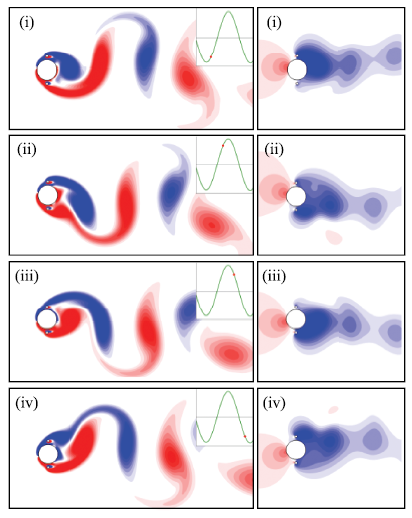Fig.10   Vorticity fields (left) and pressure fields (right) around the circular cylinder at$U_{\rm r} = 11.0$ and$\theta = 90^ \circ$

### 图11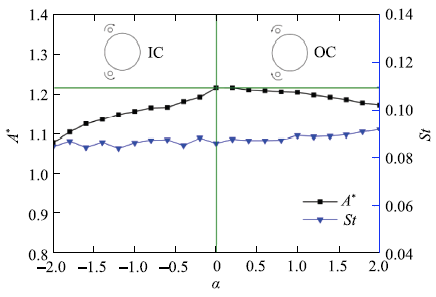Fig.11   Vibration amplitude and vortex shedding frequency versus the nondimensional rotation speed ($\alpha )$ with$\theta = 60^ \circ$ and$U_{\rm r} = 11.0$

## 4 结 论

(1)当控制角较小($\theta = 30^ \circ)$时,附加小圆柱对主圆柱的振动起到抑制作用,涡激振动的锁定区间从无附加圆柱的$3.5 \le U_{\rm r} < 8.5$缩短为$3.5 \le U_{\rm r} < 7.0$,主圆柱的最大振幅从$A^ * = 0.56$下降到$A^ * = 0.51$.

(2)当控制角为$\theta = 45^ \circ \sim 60^ \circ$时,附加小圆柱对主圆柱涡激振动的促进作用最明显.此时主圆柱振动分为两个分支,分别为初始分支和弛振分支.在弛振分支,主圆柱的脱涡频率与自然频率相接近,主圆柱的振幅随着折合流速的增大而持续增大.在$U_{\rm r}=11.0$时,最大振幅分别为$A^ * = 1.08~(\theta = 45^ \circ )$和$A^ * = 1.22~(\theta = 60^ \circ)$,比无附加圆柱工况的最大振幅($A^ * = 0.56)$分别大了93%和118%.

(3)当控制角较大($\theta = 75^ \circ \sim 90^ \circ)$时,附加小圆柱对主圆柱涡激振动的促进作用下降.此时主圆柱振动分为两个分支,分别为初始分支和下降分支.在下降分支,主圆柱的振幅随着折合流速的增加而减小,但仍比无附加圆柱工况的较大.

(4)在弛振阶段,随着折合流速的增大,主圆柱升力与位移的相位差快速增大,流体为振动圆柱输入了更多的能量,主圆柱振幅得以随着折合流速持续增加.此外,升力在弛振分支出现了3倍频分量,但由于其强度相对于基频分量较弱,因此对主圆柱振动的促进作用有限.

(5)在弛振阶段,尾涡模式为P+S模式,其中P为同向旋转的涡对,S为单个漩涡.这与高雷诺数单圆柱涡激振动P+S模式中的反向涡对有差别.

(6)控制圆柱的旋转速度对主圆柱的涡激振动振幅影响较小,当控制圆柱旋转时,无论旋转速度正负,均使主圆柱的振幅降低,但内向旋转时,振幅降低幅度更大.控制圆柱的旋转速度对旋涡的脱落频率几乎没有影响.

The authors have declared that no competing interests exist.

## 参考文献 原文顺序 文献年度倒序 文中引用次数倒序 被引期刊影响因子

Sarpkaya T .

A critical review of the intrinsic nature of vortex-induced vibrations

Journal of Fluids and Structures, 2004,19:389-447

URL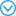This is a comprehensive review of the progress made during the past two decades on vortex-induced vibration (VIV) of mostly circular cylindrical structures subjected to steady uniform flow. The critical elements of the evolution of the ideas, theoretical insights, experimental methods, and numerical models are traced systematically; the strengths and weaknesses of the current state of the understanding of the complex fluid/structure interaction are discussed in some detail. Finally, some suggestions are made for further research on VIV.

Wu X, Ge F, Hong Y .

A review of recent studies on vortex-induced vibrations of long slender cylinders

Journal of Fluids and Structures, 2012,28:292-308

URLThis paper reviews the progress made during the past decade on vortex-induced vibration (VIV) of long slender cylindrical structures. When the aspect ratio, which is defined as the ratio of length to diameter for cylindrical structures, is large enough (10 2 10 3), some unexpected phenomena occur, e.g., dual resonance, multi-mode vibration, unsteady lock-in, the third and higher harmonic fluid forces and traveling wave dominant response, as summarized in this paper. In addition, a brief outline is given of numerical methods used in predicting the response of long slender cylinder undergoing VIV.

Williamson CHK, Govardhan R .

Vortex-induced vibrations

Annual Review of Fluid Mechanics, 2004,36:413-455

Blevins RD . Flow-Induced Vibrations. New York: Van Nostrand Reinhold, 1990

Bernitsas MM, Raghavan K, Ben-Simon Y , et al.

VIVACE (vortex induced vibration aquatic clean energy): A new concept in generation of clean and renewable energy from fluid flow

Journal of Offshore Mechanics and Arctic Engineering, ASME Transactions, 2008,130(4):041101-041115

Sakamoto H, Haniu H .

Optimum suppression of fluid forces acting in a circular cylinder

Journal of Fluids Engineering, 1994,116:221-227

Strykowski PJ, Sreenivasan KR .

On the formation and suppression of vortex `shedding' at low Reynolds numbers

Journal of Fluid Mechanics, 1990,218:71-107

URLVortex 'shedding' behind circular cylinders can be altered and suppressed altogether (or 'controlled') over a limited range of Reynolds numbers, by a proper placement of a second, much smaller, cylinder, in the near wake of the main cylinder. This new and dramatic suppression of vortex 'shedding' is the subject of this paper. Details of the phenomenon are documented through parallel experimental and numerical investigations, including flow visualisation. Temporal growth rate measurements of the velocity fluctuations reveal that the presence of the smaller cylinder reduces the growth rate of the disturbances leading to vortex 'shedding' and that its suppression, accompanied by the disappearance of sharp spectral peaks, coincides with negative temporal growth rates. It is argued that the presence of the secondary cylinder has the effect of altering the local stability of the flow by smearing and diffusing concentrated vorticity in the shear layers behind the body; a related effect is that the secondary cylinder diverts a small amount of fluid into the wake of the main cylinder. A united explanation of the formation and suppression of the vortex street is attempted, and it is suggested that the vortex 'shedding' is associated with temporally unstable eigenmodes which are heavily weighted by the near field. It is also shown that absolute instability is relevant up to a point in explaining vortex 'shedding', whose suppression can similarly be associated with altering the instability in the near wake region from absolute to convective.

Wu W, Wang J .

Numerical simulation of VIV for a circular cylinder with a downstream control rod at low Reynolds number

European Journal of Mechanics-B/Fluids, 2018,68:153-166

Schulmeister JC, Dahl JM, Weymouth GD , et al.

Flow control with rotating cylinders

Journal of Fluid Mechanics, 2017,825:743-763

URLNumerical simulations and sensitivity analysis are carried out regarding controlvortex shedding from a circular cylinder using small rotating and non-rotating control cylinders. Prediction of the changes in unsteady wake, instability growth rate, and frequency produced by the control devices is presented in the framework of sensitivity analysis to steady field force. In the case of the... [Show full abstract]

Zhu H, Yao J, Ma Y , et al.

Simultaneous CFD evaluation of VIV suppression using smaller control cylinders

Journal of Fluids and Structures, 2015,57:66-80

URL61A CFD model coupling with a FSI method is used to evaluate VIV suppression.61Placing small rods at 45° to the downstream vector can achieve a good suppression.61Rotating control cylinders with Uc=10 can further enhance VIV suppression.

Zhu H, Gao Y .

Vortex-induced vibration suppression of a main circular cylinder with two rotating control rods in its near wake: Effect of the rotation direction

Journal of Fluids and Structures, 2017,74:469-491

URLRotating small control rods in the near wake of a circular cylinder is a combination of both passive and active control techniques. The effect of rotation direction of control rods on vortex-induced vibration (VIV) suppression is investigated numerically by computational fluid dynamics (CFD) models coupling with a fluid tructure interaction (FSI) computational method. Two small rods with diameter ratio of 0.06 are located symmetrically at 135 right behind the main cylinder, and the gap between the main cylinder and control rods is 0.09 D . The Reynolds number and the reduced velocity are 3484 and 6.0, respectively. Five different rotation modes are considered including the no-rotating case. The results indicate that the presence of control rods changes the momentum and kinetic energy distribution. Placing stationary rods in this specific position can achieve a good suppression effect, and the effect is enhanced when the control rods are inward counter rotating. Inward counter-rotating rods facilitate the momentum injection from the outer flow into the boundary layer, resulting in the delay of boundary layer separation and the shift of separation point. Therefore, the wake becomes narrower. However, control rods rotating outwardly play a counterproductive role in flow control and the vibration is enhanced. Rotating rods upwardly and downwardly have almost the same vibration responses. The cross-flow vibration is suppressed while the in-line vibration is enhanced at these two mirrored cases.

An active flow control strategy for the suppression of vortex structures behind a circular cylinder

European Journal of Mechanics-B/Fluids, 2010,29:93-104

URLAn algorithm is proposed to model, predict and control vortex shedding behind a circular cylindrical configuration. The main ingredients of the algorithm include multiple-feedback sensors, actuators (with zero net mass injection) and a control strategy. Along with the mass and momentum conservation equations, a control equation is implemented to enable the desired flow control goals. A number of sensors are chosen in the downstream of the body to report the state of the flow. The role of externally controllable actuators on the fluid flow patterns past a circular configuration is assessed. To enable, zero net mass injection, two simple rotary type mechanical actuators are located at 120°, right behind the main cylinder. The popular finite volume based SIMPLE scheme is employed for the numerical calculations. As a precursor, the scheme simulates flow past an isolated cylinder, which is validated over a moderate range of Reynolds numbers. The design parameters of interest such as Strouhal number, drag and lift coefficients etc are used for the purpose of validation. The simulated flow fields are compared against the flow visualization study, which clearly demonstrates the efficacy of the actuators at discrete levels of rotation. The basic character of the flow is completely modified at U c/ U ∞02=022.0 and Re = 100, where a complete suppression of vortex shedding is observed. This is tantamount to complete control of all the global instability modes. Fictitious tracer particles are released to visualize the vortex structures in the form of streaklines. The results clearly demonstrate the effectiveness of a rather simple active control algorithm in suppressing the vortex structures. All the relevant fluid flow features of the bluff-body fluid mechanics under the influence of actuators are studied in the sub-critical Reynolds number range of Re = 100–300.

URL     Magsci( Lee Chunhian, Peng Shaobo, Wu Ziniu .

Numerical study of flow around a main cylinder by controlled satellite cylinders

Journal of Beijing University of Aeronautics and Astronautics, 2003,29(11):951-958 (in Chinese))

URL     MagsciJiménez-González JI, Huera-Huarte FJ .

Experimental sensitivity of vortex-induced vibrations to localized wake perturbations

Journal of Fluids and Structures, 2017,74:53-63

URLAs a validation of the sensitivity maps, we demonstrate how by using control cylinders with diameters of only 12% of the main cylinder diameter, reductions of VIV response of more than 65%, can be reached. The use of Digital Particle Image Velocimetry (DPIV) has allowed us to identify the physical mechanisms underlying the VIV response modifications induced by the control cylinders.

Korkischko I, Meneghini JR .

Suppression of vortex-induced vibration using moving surface boundary-layer control

Journal of Fluids and Structures, 2012,34:259-270

URLExperimental results of flow around a circular cylinder with moving surface boundary-layer control (MSBC) are presented. Two small rotating cylinders strategically located inject momentum in the boundary layer of the cylinder, which delays the separation of the boundary layer. As a consequence, the wake becomes narrower and the fluctuating transverse velocity is reduced, resulting in a recirculation free region that prevents the vortex formation. The control parameter is the ratio between the tangential velocity of the moving surface and the flow velocity (Uc/U). The main advantage of the MSBC is the possibility of combining the suppression of vortex-induced vibration (VIV) and drag reduction. The experimental tests are preformed at a circulating water channel facility and the circular cylinders are mounted on a low-damping air bearing base with one degree-of-freedom in the transverse direction of the channel flow. The mass ratio is 1.8. The Reynolds number ranges from 1600 to 7500, the reduced velocity varies up to 17, and the control parameter interval is Uc/U=5 10. A significant decreasing in the maximum amplitude of oscillation for the cylinder with MSBC is observed. Drag measurements are obtained for statically mounted cylinders with and without MSBC. The use of the flow control results in a mean drag reduction at Uc/U=5 of almost 60% compared to the plain cylinder. PIV velocity fields of the wake of static cylinders are measured at Re=3000. The results show that the wake is highly organized and narrower compared to the one observed in cylinders without control. The calculation of the total variance of the fluctuating transverse velocity in the wake region allows the introduction of an active closed-loop control. The experimental results are in good agreement with the numerical simulation studies conducted by other researchers for cylinders with MSBC.

Silva-Ortega M, Assi GRS .

Hydrodynamic loads on a circular cylinder surrounded by two, four and eight wake-control cylinders

Ocean Engineering, 2018,153:345-352

Lou M, Chen P, Chen Z .

Experimental investigation on the suppression of vortex-induced vibration of two interfering risers by control rods

Ships and Offshore Structures, 2017,12(8):1117-1126

Zhu H, Yao J .

Numerical evaluation of passive control of VIV by small control rods

Applied Ocean Research, 2015,51:93-116

URLHighlights 61 A combined FSI–CFD model is used to conduct evaluation of VIV suppression. 61 Effects of rod number, diameter ratio, gap ratio and Reynolds number are discussed. 61 A comprehensive evaluation is performed based on VIV suppression and the cost of inputs. 61 The attachment with 9 rods with d/D&nbsp;=&nbsp;0.15 and G/D&nbsp;=&nbsp;0.6 can achieve a good VIV effect. Abstract Flow past a circular cylinder with multiple small control rods is studied by numerical simulation for ReD ranging from 1161.3 to 6387.1. The Reynolds-Averaged-Navier–Stokes (RANS) equations and shear stress transport (SST) k&nbsp;61&nbsp;ω turbulence model are used to calculate the vortex field, while a fourth-order Runge–Kutta method is employed for evaluating the structure dynamics of the cylinder group. Comparisons with experimental results demonstrate the validation of this method. This study is concerned with the vortex induced vibration (VIV) suppression efficacy of small control rods placed around a main cylinder. The effects of control rod number, diameter ratio, spacing ratio and Reynolds number on the hydrodynamics and vibration responses of the main cylinder are investigated. The reduced percents of in-line and cross-flow amplitudes and the increased percents of the whole cross-sectional area of cylinders and the drag coefficient are used to give a comprehensive evaluation. Results of simulation indicate that placing small rods with appropriate number at appropriate locations can achieve good suppression effectiveness at a wide range of Reynolds number. The numerical result for the case with nine control rods, diameter ratio of 0.15 and spacing ratio of 0.6 shows the best suppression effect among the cases investigated in this study.

Silva-Ortega M, Assi GRS .

Suppression of the vortex-induced vibration of a circular cylinder surrounded by eight rotating wake-control cylinders

Journal of Fluids and Structures, 2017,74:401-412

URLThe present work investigates the use of a polar array of 8 wake-control cylinders as a means of suppressing the vortex-induced vibration (VIV) of a larger circular cylinder. The diameter of the control cylinders and their rotation speed were the main parameters investigated. Experiments have been performed in water at Reynolds numbers between 5000 and 50,000. The rotating cylinders suppressed the peak amplitude of displacement by around 70% when compared to that of a bare cylinder. A similar response was obtained even if the rotation speed of the control cylinders was kept constant in relation to the flow speed. A specific configuration with 8 non-rotating control cylinders achieved an even better 99% suppression. As a consequence of reduced vibrations, the fluctuation of lift and mean drag were not as amplified due to VIV. The results pave the way for further studies concerning system optimization and support the development of efficient VIV suppressors and dynamic positioning systems for large floating offshore platforms and other applications.

Song Z, Duan M, Gu J .

Numerical investigation on the suppression of VIV for a circular cylinder by three small control rods

Applied Ocean Research, 2017,64:169-183

URLLaminar flow past a circular cylinder with 3 small control rods is investigated by numerical simulation. This study is concerned with the suppression efficacy of vortex induced vibration by small control rods located around a main cylinder. The effects of the attack angle and rod-to-cylinder gap ratio on the hydrodynamics and vibration responses of the main cylinder are investigated. The attack angle of α 02=0245° is performed as the critical angle for VIV suppression of 3 control rods. The 3 control rods have no effect on VIV suppression when the attack angle is less than the critical angle. The 3 control rods have an excellent VIV suppression efficacy when the attack angle is larger than the critical angle. The transverse vibration frequency of the cylinder with 3 control rods is less than that for an isolated cylinder for all the configurations. The numerical results for the configurations of α 02=0245° & 60°, G / D 02=020.6–1.2 show excellent suppression efficient among the cases investigated in this study. The best suppression efficient is found at α 02=0245°, G / D 02=020.9 for 3 control rods. 2 rods in behind of the main cylinder perform more efficient than that of 1 rod in front for VIV suppression as the gap ratio of G / D less than 1.0.

( Wu Hao . Experimental and numerical studies on the suppression of vortex induced vibration of long flexible riser by multiple control rods. [PhD Thesis]. Da Lian: Dalian University of Technology, 2013 (in Chinese))

URL( Song Jining, Lin, Zhang Jianqiao , et al.

Experimental investigation of suppression of vortex-induced vibration of marine risers by three control rods

The Ocean Engineering, 2009,27(3):23-29 (in Chinese))

URLURL( Lou Min, Zhu Yan .

Experimental study on vortex-induced vibration suppression of tandem risers with three-control-rods

Ship & Ocean Engineering, 2018,47(1):124-128 (in Chinese))

URLJi C, Munjiza A, Williams JJR .

A novel iterative direct-forcing immersed boundary method and its finite volume applications

Journal of Computational Physics, 2012,231:1797-1821

Chen W, Ji C, Xu W , et al.

Response and wake patterns of two side-by-side elastically supported circular cylinders in uniform laminar cross-flow

Journal of Fluids and Structures, 2015,55:218-236

URLVortex-induced vibrations (VIV) of two side-by-side elastically supported circular cylinders in a uniform flow with the Reynolds number of 100 are numerically investigated by using the immersed boundary method. The cylinders are constrained to oscillate in the cross-flow direction with a center-to-center spacing ratio T/D ranging from 2 to 5. The structural damping is set to zero to enable large vibration amplitudes in the range of reduced velocity Ur=3 10. It is found that the proximity of the cylinders does not have a significant impact to the lock-in region and cylinder responses, except at a small spacing ratio of T/D=2. The critical spacing ratio is determined as T/D=4 and beyond that the interaction between the cylinders is negligible. The following six near-wake patterns are observed; the irregular pattern, in-phase flip-flopping pattern, out-of-phase flip-flopping pattern, in-phase-synchronized pattern, antiphase-synchronized pattern and the biased antiphase-synchronized pattern. These patterns are plotted in a plane of Ur and T/D, together with approximate borderlines to distinguish one region from the others. The time histories, spectral features and wavelet transform contours of drag and lift forces are presented to elucidate the mechanisms of the in-phase and out-of-phase flip-flopping phenomena. It is established that the in-phase flip-flopping stems from the long-short near-wake pattern and its low-frequency flip-over, whereas the out-of-phase pattern originates from the large vortex shedding from the fictitious bluff-body with an augmented characteristic length.

Bourguet R, Jacono DL .

Flow-induced vibrations of a rotating cylinder

Journal of Fluid Mechanics, 2014,740:342-380

URLThe flow-induced vibrations of a circular cylinder, free to oscillate in the cross-flow direction and subjected to a forced rotation about its axis, are analysed by means of two- and three-dimensional numerical simulations. The impact of the symmetry breaking caused by the forced rotation on the vortex-induced vibration (VIV) mechanisms is investigated for a Reynolds number equal to $100$ , based on the cylinder diameter and inflow velocity. The cylinder is found to oscillate freely up to a rotation rate (ratio between the cylinder surface and inflow velocities) close to $4$ . Under forced rotation, the vibration amplitude exhibits a bell-shaped evolution as a function of the reduced velocity (inverse of the oscillator natural frequency) and reaches $1.9$ diameters, i.e. three times the maximum amplitude in the non-rotating case. The free vibrations of the rotating cylinder occur under a condition of wake body synchronization similar to the lock-in condition driving non-rotating cylinder VIV. The largest vibration amplitudes are associated with a novel asymmetric wake pattern composed of a triplet of vortices and a single vortex shed per cycle, the ${\rm T} + {\rm S}$ pattern. In the low-frequency vibration regime, the flow exhibits another new topology, the U pattern, characterized by a transverse undulation of the spanwise vorticity layers without vortex detachment; consequently, free oscillations of the rotating cylinder may also develop in the absence of vortex shedding. The symmetry breaking due to the rotation is shown to directly impact the selection of the higher harmonics appearing in the fluid force spectra. The rotation also influences the mechanism of phasing between the force and the structural response.

Shiels D, Leonard A, Roshko A .

Flow-induced vibration of a circular cylinder at limiting structural parameters

Journal of Fluids and Structures, 2001,15:3-21

URLTransverse oscillation of a dynamically supported circular cylinder in a flow at Re=100 has been numerically simulated using a high-resolution viscous-vortex method, for a range of dynamical parameters. At the limiting case with zero values of mass, damping and elastic force, the cylinder oscillates sinusoidally at amplitudeA /D=0·47 and frequency fD/U∞=0·156. For zero damping, the effects of mass and elasticity are combined into a new, “effective” dynamic parameter, which is different from the classic “reduced velocity”. Over a range of this parameter, the response exhibits oscillations at amplitudes up to 0·6 and frequencies between 0·15 and 0·2. From this response function, the classic response in terms of reduced velocity can be obtained for fixed values of the cylinder/fluid ratio m*. It displays “lock-in” at very high values of m*.

Chen W, Ji C, Wang R , et al.

Flow-induced vibrations of two side-by-side circular cylinders: Asymmetric vibration, symmetry hysteresis and near-wake patterns

Ocean Engineering, 2015,110:244-257

URLThe immersed boundary method was utilised to numerically investigate the flow-induced vibrations (FIV) of two elastically mounted side-by-side circular cylinders in a uniform flow with low Reynolds numbers. Six distinct near-wake patterns were observed; the irregular (IR) pattern, the in-phase synchronized (IS) pattern, the anti-phase synchronized (AS) pattern, the biased anti-phase synchronized (BAS) pattern, the out-of-phase flip-flopping (OFF) pattern, and the hybrid (HB) pattern. A detailed analysis on the asymmetric vibration and symmetry hysteresis phenomena was conducted by focusing on the near-wake patterns and the interaction between the cylinders. Results show that the asymmetric vibrations of the cylinders are closely related with the stably biased gap flow and the resulting narrow-wide near-wake pattern. While the symmetry hysteresis is caused by the coexistence of two distinct near-wake patterns the IS and the BAS patterns. The transition processes of BAS to IS and IS to BAS were illustrated by using the long-time histories of the lift coefficients, the combined lift coefficient and the phase differences of lift and displacement. Results on hydrodynamic forces and the vibration responses show that the HB pattern is a combination of IS, OFF and AS patterns with a very long period.

Chen W, Ji C, Williams J , et al.

Vortex-induced vibrations of three tandem cylinders in laminar cross-flow: vibration response and galloping mechanism

Journal of Fluids and Structures, 2018,78:215-238

URLURL( Chen Weilin, Ji Chunning, Xu Dong .

Galloping in vortex-induced vibration of three tandem cylinders at low Reynolds numbers and its influencing factors

Chinese Journal of Theoretical and Applied Mechanics, 2018,50(4):766-775 (in Chinese))

URLURL( Ji Chunning, Hua Yang, Xu Dong , et al.

Numerical simulation of vortex-induced vibration of a flexible cylinder exposed to shear flow at different shear rates

Chinese Journal of Theoretical and Applied Mechanics, 2018,50(1):21-31 (in Chinese))

URLURL     Magsci<p>对雷诺数<em>Re</em> = 100 间距比<em>s/D</em> = 2.5 和5.0 的并列双圆柱流致振动进行了数值模拟研究, 其中圆柱质量比<em>m</em> = 2.0, 折合流速<em>U</em><sub><em>r</em></sub> 在2.0~10.0 之间, 两圆柱仅能做横流向振动. 研究发现, 当间距比<em>s/D</em> = 2.5 时, 在折合流速4.4 &lt; <em>U</em><sub><em>r</em></sub>&lt; 4.8区间内, 两圆柱流致振动响应出现不对称振动现象, 在折合流速4.4 &lt; <em>U</em><sub><em>r</em></sub>&lt; 4.8 区间内, 两圆柱流致振动响应出现对称性迟滞现象; 而当间距比<em>s/D</em> = 2.5时, 圆柱流致振动响应与单圆柱涡激振动响应相似, 没有出现不对称振动和对称性迟滞现象. 在不对称振动区间内, 两圆柱的升、阻力参数也出现了不相等的情况. 此外, 当两圆柱不对称振动时, 圆柱间隙流稳定地偏斜向其中的一个圆柱; 相应地, 尾涡也出现了宽窄不等的模式. 窄尾流圆柱的振幅和升、阻力均较宽尾流圆柱的大. 通过对比不对称振动现象发生前后的尾涡模式, 对新现象的产生机制进行了阐述.</p>

( Chen Weilin, Ji Chunning, Xu Wanhai .

Numerical investigation on the asymmetric vibration and symmetry hysteresis of flow-induced vibration of two side-by-side cylinders

Chinese Journal of Theoretical and Applied Mechanics, 2015,47(5):731-739 (in Chinese))

URL     Magsci<p>对雷诺数<em>Re</em> = 100 间距比<em>s/D</em> = 2.5 和5.0 的并列双圆柱流致振动进行了数值模拟研究, 其中圆柱质量比<em>m</em> = 2.0, 折合流速<em>U</em><sub><em>r</em></sub> 在2.0~10.0 之间, 两圆柱仅能做横流向振动. 研究发现, 当间距比<em>s/D</em> = 2.5 时, 在折合流速4.4 &lt; <em>U</em><sub><em>r</em></sub>&lt; 4.8区间内, 两圆柱流致振动响应出现不对称振动现象, 在折合流速4.4 &lt; <em>U</em><sub><em>r</em></sub>&lt; 4.8 区间内, 两圆柱流致振动响应出现对称性迟滞现象; 而当间距比<em>s/D</em> = 2.5时, 圆柱流致振动响应与单圆柱涡激振动响应相似, 没有出现不对称振动和对称性迟滞现象. 在不对称振动区间内, 两圆柱的升、阻力参数也出现了不相等的情况. 此外, 当两圆柱不对称振动时, 圆柱间隙流稳定地偏斜向其中的一个圆柱; 相应地, 尾涡也出现了宽窄不等的模式. 窄尾流圆柱的振幅和升、阻力均较宽尾流圆柱的大. 通过对比不对称振动现象发生前后的尾涡模式, 对新现象的产生机制进行了阐述.</p>

Qin B, Alam MM, Zhou Y .

Two tandem cylinders of different diameters in cross-flow: Flow-induced vibration

Journal of Fluid Mechanics, 2017,829:621-658Abstract This paper presents a systematic study of the cross-flow-induced vibration on a spring-supported circular cylinder of diameter $D$ placed in the wake of a fixed cylinder of smaller diameter $d$ . The ratios $d/D$ and $L/d$ are varied from 0.2 to 1.0 and from 1.0 to 5.5, respectively, where $L$ is the distance between the centre of the upstream cylinder to the forward stagnation point of the downstream cylinder. Extensive measurements are conducted to capture the cylinder vibration and frequency responses, surface pressure, shedding frequencies and flow fields using laser vibrometer, hot-wire, pressure scanner and particle image velocimetry techniques. Six distinct flow regimes are identified. It has been found that a violent vibration may erupt for the spring-supported cylinder, and its dependence on $d/D$ and $L/d$ is documented. A careful examination and analysis of the flow structure, along with the simultaneously captured pressure distribution around and vibration of the downstream cylinder, cast light upon the mechanisms behind this vibration and its sustainability. The roles of added mass, flow-induced damping and physical aspects in the process of initiating the vibration are discussed in detail.

Williamson CHK, Roshko A .

Vortex formation in the wake of an oscillating cylinder

Journal of Fluids and Structures, 1988,2:355-381

URLWhen a body oscillates laterally (cross-flow) in a free stream, it can synchronize the vortex formation frequency with the body motion frequency. This fundamental “lock-in” regions is but one in a whole series of synchronization regions, which have been found in the present paper, in an amplitude-wavelength plane (defining the body trajectory) up to amplitudes of five diameters. In the fundamental region, it is shown that the acceleration of the cylinder each half cycle induces the roll-up of the two shear layers close to the body, and thereby the formation of four regions of vorticity each cycle. Below a critical wavelength, each half cycle sees the coalescence of a pair of like-sign vortices and the development of a Karman-type wake. However, beyond this wavelength the like-sign vortices convect away from each other, and each of them pairs with an opposite-sign vortex. The resulting wake comprises a system of vortex pairs which can convect away from the wake centerline. The process of pairing causes the transition between these modes to be sudden, and this explains the sharp change in the character of the cylinder forces observed by Bishop and Hassan, and also the jump in the phase of the lift force relative to body displacement. At precisely the critical wavelength, only two regions of vorticity are formed, and the resulting shed vorticity is more concentrated than at other wavelengths. We interpret this particular case as a condition of “resonant synchronization”, and it corresponds with the peak in the body forces observed in Bishop and Hassan's work.

Paidoussis MP, Price SJ , et al. Fluid-Structure Interactions: Cross-Flow-Induced Instabilities. Cambridge University Press. 2010

Navrose N, Mittal S .

Lock-in in vortex-induced vibration

Journal of Fluid Mechanics, 2016,794:565-594

URLThe phenomenon of lock-in in vortex-induced vibration of a circular cylinder is investigated in the laminar flow regime ( ). Direct time integration (DTI) and linear stability analysis (LSA) of the governing equations are carried out via a stabilized finite element method. Using the metrics that have been proposed in earlier studies, the lock-in regime is identified from the results of DTI. The LSA yields the eigenmodes of the coupled fluid090009structure system, the associated frequencies ( ) and the stability of the steady state. A linearly unstable system, in the absence of nonlinear effects, achieves large oscillation amplitude at sufficiently large times. However, the nonlinear terms saturate the response of the system to a limit cycle. For subcritical , the occurrence of lock-in coincides with the linear instability of the fluid090009structure system. The critical is the Reynolds number beyond which vortex shedding ensues for a stationary cylinder. For supercritical , even though the aeroelastic system is unstable for all reduced velocities ( ) lock-in occurs only for a finite range of . We present a method to estimate the time beyond which the nonlinear effects are expected to be significant. It is observed that much of the growth in the amplitude of cylinder oscillation takes place in the linear regime. The response of the cylinder at the end of the linear regime is found to depend on the energy ratio, , of the unstable eigenmode. is defined as the fraction of the total energy of the eigenmode that is associated with the kinetic and potential energy of the structure. DTI initiated from eigenmodes that are linearly unstable and whose energy ratio is above a certain threshold value lead to lock-in. Interestingly, during lock-in, the oscillation frequency of the fluid090009structure system drifts from towards a value that is closer to the natural frequency of the oscillator in vacuum ( ). In the event of more than one eigenmode being linearly unstable, we investigate which one is responsible for lock-in. The concept of phase angle between the cylinder displacement and lift is extended for an eigenmode. The phase angle controls the direction of energy transfer between the fluid and the structure. For zero structural damping, if the phase angle of all unstable eigenmodes is less than 9000°, the phase angle obtained via DTI evolves to a value that is close to 000°. If, on the other hand, the phase angle of any unstable eigenmode is more than 9000°, it settles to 18000°, approximately in the limit cycle. A new approach towards classification of modes is presented. The eigenvalues are tracked over a wide range of while keeping and mass ratio ( ) fixed. In general, for large values of , the eigenmodes corresponding to the two leading eigenvalues exhibit a decoupled behaviour with respect to . They are classified as the fluid and elastic modes. However, for relatively low such a classification is not possible. The two leading modes are coupled and are referred to as fluid090009elastic modes. The regime of such occurrence is shown on the parameter space.

/

 〈〉